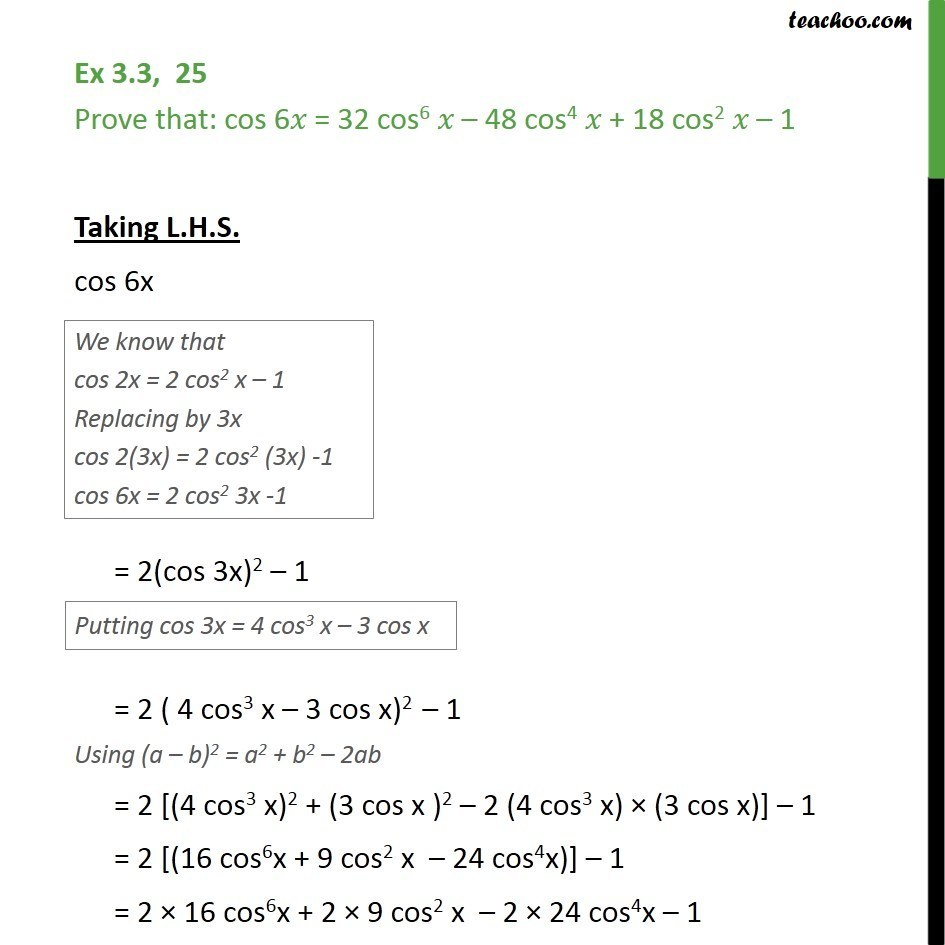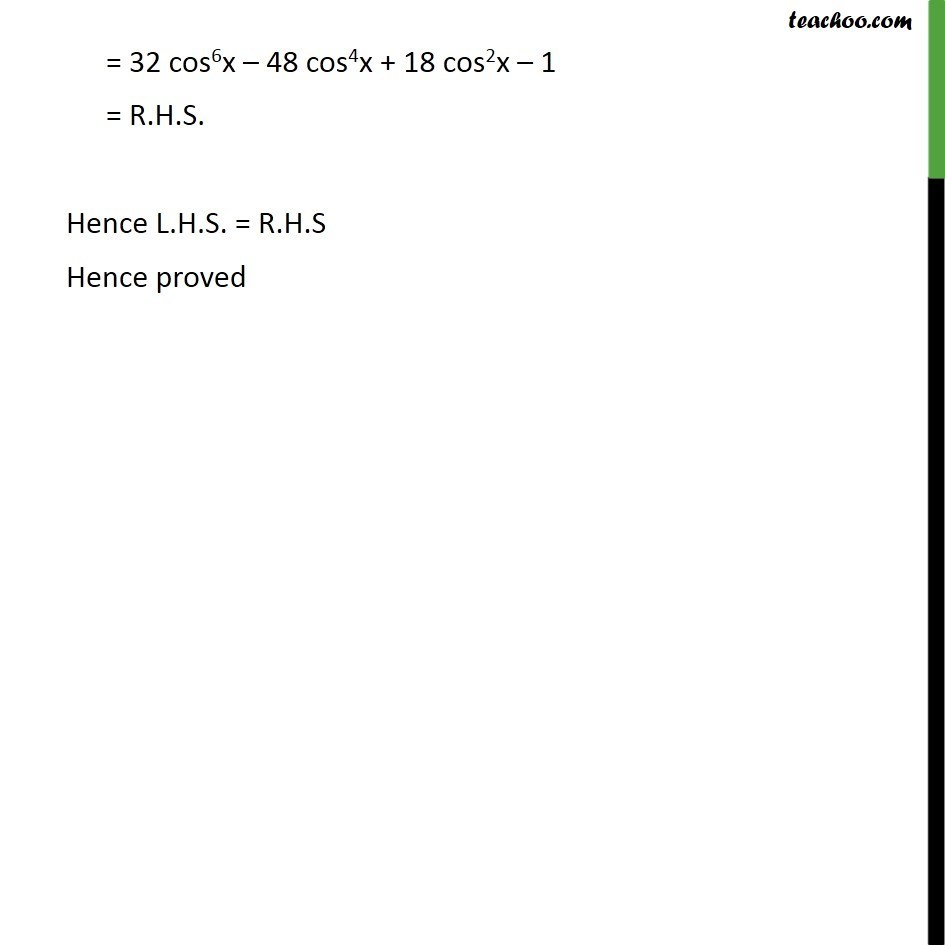Learn All Concepts of Chapter 2 Class 11 Relations and Function - FREE. Check - Trigonometry Class 11 - All Concepts1. Chapter 3 Class 11 Trigonometric Functions
2. Serial order wise
3. Ex 3.3

Transcript

Ex 3.3, 25 Prove that: cos 6 = 32 cos6 48 cos4 + 18 cos2 1 Taking L.H.S. cos 6x = 2(cos 3x)2 1 = 2 ( 4 cos3 x 3 cos x)2 1 Using (a b)2 = a2 + b2 2ab = 2 [(4 cos3 x)2 + (3 cos x )2 2 (4 cos3 x) (3 cos x)] 1 = 2 [(16 cos6x + 9 cos2 x 24 cos4x)] 1 = 2 16 cos6x + 2 9 cos2 x 2 24 cos4x 1 = 32 cos6x 48 cos4x + 18 cos2x 1 = R.H.S. Hence L.H.S. = R.H.S Hence proved

Ex 3.3